# System of Linear Equations

Before you we try to understand the system of linear equations, we must understand the basic terminologies that we are going to use in the linear algebra.

### Linear Equations

Linear equation represents a straight line on a xy-plane or co-ordinate plane.

a_{1}x + a_{2}y = b
• The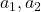are real number constants and both cannot be zero at the same time.
• The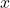and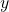are called variables. Hence, the equation is linear equation in variableand.

Therefore, linear equation in n variable is given as

a_{1}x_{1} + a_{2}x_{2} + a_{3}x_{3} + \dots + a_{n}x_{n} = b

where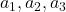and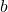are real number constants.

#### Examples of linear equations

Here are some examples of linear equations.

\begin{aligned}
3x + 5 = 13\\ \\
\frac{3}{4}y + 3z = 1
\end{aligned}

From the above examples, note that linear equations show following characteristics.

• There is not term in the equation with root or product.
• All variables are in power of 1.
• They do not appear as an argument of another function.

Here are examples of non-linear equations to help you understand the basic differences.

\begin{aligned}
&2 + \sqrt{y} = 7\\
&x + 2yz + z = 6\\
&sin \hspace{3px} x + cos \hspace{3px}x = 1
\end{aligned}

### Solution Of Linear Equations

A linear equation

a_{1}x_{1} + a_{2}x_{2} + ... + a_{n}x_{n}

has a sequence of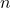numbers

c_{1},c_{2},..., c_{n}

as solution which satisfy the equation once we substitute them with the variables.

For example,

a_{1}x_{1} + a_{2}x_{2} + a_{2}x_{2} + ... + a_{n}x_{n]} = b\\ \\


The solution is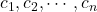Then,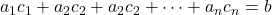will satisfy the equation.

The set of all solutions of a linear equation is called solution set or general solution to the linear equation.

#### How to find solution to linear equations ?

Now we will solve two linear equation using substitute method.

Problem #1 : solve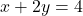.

Solution:

We can solve like ordinary algebraic equation. Solve for x for solve for y.

Add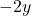to both side of the equation

x + 2y - 2y = 4 - 2y

now we have

x = 4 - 2y

Let’s give an arbitrary value to y. This way we get value for.

 \begin{aligned}
&y = 1\\
&x = 4 - 2y\\
&x = 4 - 2\\
&Therefore, \hspace{2mm}x = 2
\end{aligned}

Problem #2 : solve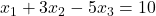——————————————————————————-

Solution:
————–
This time we can solve for two variables and get the third. We get following equations.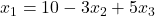Let us give arbitrary value to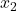and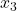.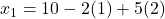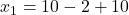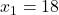### System of Linear Equations

The system of linear equations is a set of linear equations withvariables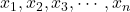.

\begin{aligned}
&x_{1} + 5x_{2} + 2x_{3} = 6\\
&3x_{1} + x_{2} - x_{3} = 4\\
&4x_{1} + 2x_{2} + 2x_{3} = 6
\end{aligned}

The solution set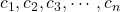when replaced with variables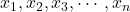will satisfy every equation in the linear system. In the above system of linear equations,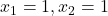and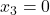will satisfy all the equations.

In the next post, we will discuss about inconsistent and consistent system of linear equations.